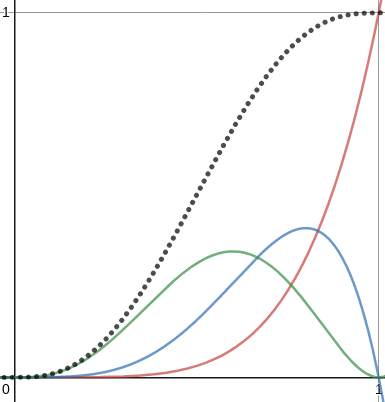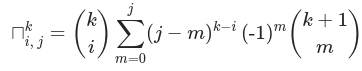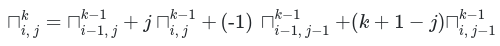As per usual, I was reading an article. This time it was “Understanding Perlin Noise” by Adrian Biagioli. Perlin noise is an algorithm to generate a smooth cloud-like noise. It is very useful and can look like this: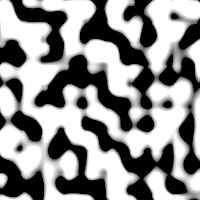At some point in the article, it mentions that Perlin noise uses easing functions to achieve that smoothness. And it tells you that improved version of Perlin noise uses 6t⁵-15t⁴+10t³ as its easing function which looks like this (source):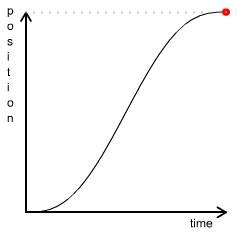So I was wondering where they got that function. Turns out, the answer is simple, it’s derived from Bézier curves. It’s the curve you get when you interpolate in 1D between 6 points. Those six points are in order: 0, 0, 0, 1, 1, 1.

Intuitively each point will attract the curve to itself. So the first three points attract the curve to 0, which is why it starts out slow, and the last three points attract the curve to 1, thus flattening it out.

To get the formula, we sum multiply each Bézier function with the point’s value, and sum them all. f₀ to f₅ are the Bernstein polynomials of degree 5.

Bernstein polynomials of degree 5. f₀: orange, f₁: black, f₂: purple, f₃: green, f₄: blue and f₅: red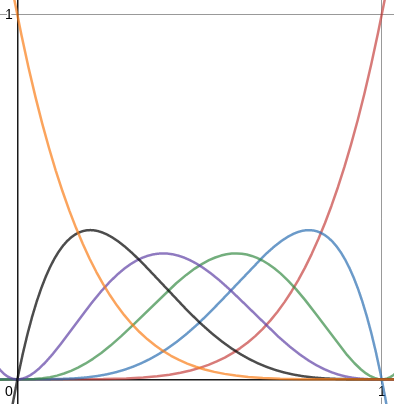Since the first 3 points are at 0, we only sum the last 3 functions. In green f₃ = 10(t⁵ - 2t⁴ + t³), in blue f₄ = 5(-t⁵ + t⁴), and in blue f₅ = t⁵. The sum is our expected 6t⁵ - 15t⁴ + 10t³ in black.